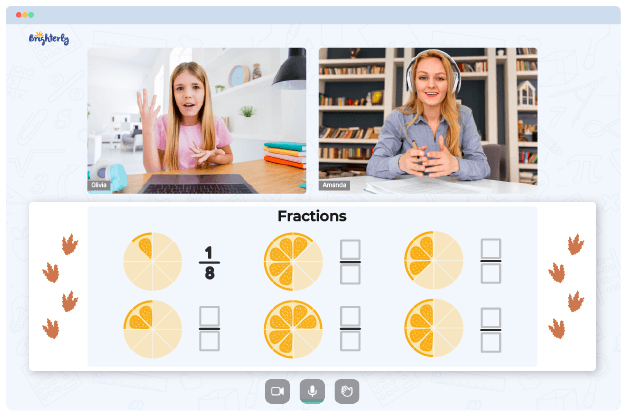# Adding And Subtracting Fractions With Unlike Denominators Worksheets

Addition and subtraction of fractions using unlike denominators require attention to detail, patience, and practice on the side of both teacher and student. However, with the consistent use of adding and subtracting fractions with unlike denominators worksheets, children can practice and learn how to solve math problems. This piece describes the benefits and the ways to find these worksheets.

## Benefits of Using an Adding and Subtracting Fractions with Unlike Denominators Worksheet

Using an adding and subtracting fractions with unlike denominators worksheet can be beneficial to a child in many ways. Here are some of these benefits:

### The worksheets come with fun visuals

Kids are easily distracted, and teaching them requires some extra drama, which in some cases could be colorful paper booklets. If you want children to add and subtract fractions with unlike denominators successfully, you need to ensure that they enjoy the learning process. By using an add and subtract fractions with unlike denominators worksheet with colorful fun visuals, you may just hit that teaching success you want.

Math for Kids

Is Your Child Struggling With Math?
1:1 Online Math Tutoring### The worksheets help to learn how to solve math problems quickly

Adding and subtracting fractions with unlike denominators worksheets contain numerous practice questions. Students must go through the exercises, learning to solve them with little supervision.

With every solution they create and every answer they get right, they get better at solving the problems and learn how to do it faster. More importantly, they can use this newly acquired math-solving speed to handle real-life math-needing situations, which is the basis of all foundational math lessons.### Adding And Subtracting Fractions Worksheets PDF

Adding And Subtracting Fractions With Unlike Denominators Worksheet### Adding And Subtracting Fractions Worksheets PDF

Add And Subtract Fractions With Unlike Denominators Worksheet### Adding And Subtracting Fractions Worksheets PDF

Adding And Subtracting Fractions With Unlike Denominators Worksheets Pdf### Adding And Subtracting Fractions Worksheets PDF

Adding And Subtracting Fractions With Unlike Denominators Word Problems Worksheets

You can download adding and subtracting fractions with unlike denominators worksheets in PDF on math websites and share them with your kids. You can also get adding and subtracting fractions with unlike denominators word problems worksheets depending on what part of the lessons you teach.

Final tip: Ensure that you are downloading worksheets with fun visuals to aid your teaching process and kids’ understanding of the math problems on the worksheet.

### Fractions Worksheets

Need help with Fractions?• Start studying with an online tutor.

Is your child finding it challenging to grasp fractions lessons? An online tutor could be the solution.